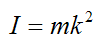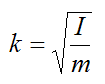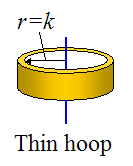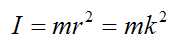Sometimes the moment of inertia of a body about a specified axis will be given using the radius of gyration, k. Given the value k and the mass of the body (m) we can calculate its moment of inertia (I), using the following formula:Consequently, the radius of gyration is given byThe physical interpretation of the radius of gyration is that it is the radius of a uniform thin hoop (or ring), having the same moment of inertia (about an axis passing through its geometric center – shown below), as the given body about the specified axis.The moment of inertia of the thin hoop about the blue axis passing through its geometric center is:The radius of gyration can be useful for listing in a table. If you want to know the moment of inertia of a complex shaped body about a given axis you simply look up its radius of gyration, and then (knowing its mass) apply the above formula to find the moment of inertia.